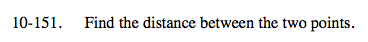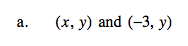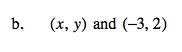### Home > A2C > Chapter 10 > Lesson 10.2.6 > Problem10-151

10-151.Notice that the y values are the same. (the line is horizontal)

Just subtract −3 from x to find the distance.

x + 3Use the distance formula.

$d=\sqrt{(x_{1}-x_{2})^{2}+(y_{1}-y_{2})^{2}}$

$\sqrt{(x+3)^{2}+(y-2)^{2}}$